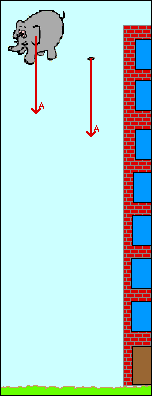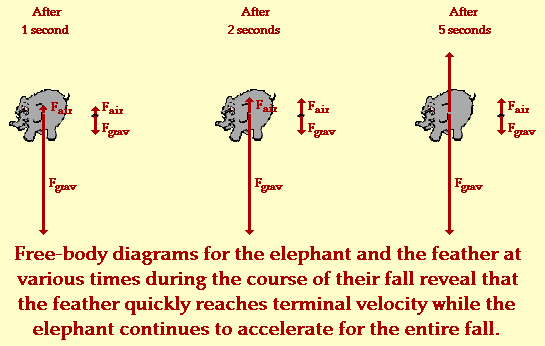Elephant and Feather - Air ResistanceSuppose that an elephant and a feather are dropped off a very tall building from the same height at the same time. We will assume the realistic situation that both feather and elephant encounter air resistance. Which object - the elephant or the feather - will hit the ground first? The animation at the right accurately depicts this situation. The motion of the elephant and the feather in the presence of air resistance is shown. Further, the acceleration of each object is represented by a vector arrow.

Most people are not surprised by the fact that the elephant strikes the ground before the feather. But why does the elephant fall faster? This question is the source of much confusion (as well as a variety of misconceptions). Test your understanding by making an effort to identify the following statements as being either true or false.

TRUE or FALSE:

1. The elephant encounters a smaller force of air resistance than the feather and therefore falls faster.
2. The elephant has a greater acceleration of gravity than the feather and therefore falls faster.
3. Both elephant and feather have the same force of gravity, yet the acceleration of gravity is greatest for the elephant.
4. Both elephant and feather have the same force of gravity, yet the feather experiences a greater air resistance.
5. Each object experiences the same amount of air resistance, yet the elephant experiences the greatest force of gravity.
6. Each object experiences the same amount of air resistance, yet the feather experiences the greatest force of gravity.
7. The feather weighs more than the elephant, and therefore will not accelerate as rapidly as the elephant.
8. Both elephant and feather weigh the same amount, yet the greater mass of the feather leads to a smaller acceleration.
9. The elephant experiences less air resistance and than the feather and thus reaches a larger terminal velocity.
10. The feather experiences more air resistance than the elephant and thus reaches a smaller terminal velocity.
11. The elephant and the feather encounter the same amount of air resistance, yet the elephant has a greater terminal velocity.

If you answered TRUE to any of the above questions, then perhaps you have some confusion about either the concepts of weight, force of gravity, acceleration of gravity, air resistance and terminal velocity. The elephant and the feather are each being pulled downward due to the force of gravity. When initially dropped, this force of gravity is an unbalanced force. Thus, both elephant and feather begin to accelerate (i.e., gain speed). As the elephant and the feather begin to gain speed, they encounter the upward force of air resistance. Air resistance is the result of an object plowing through a layer of air and colliding with air molecules. The more air molecules which an object collides with, the greater the air resistance force. Subsequently, the amount of air resistance is dependent upon the speed of the falling object and the surface area of the falling object. Based on surface area alone, it is safe to assume that (for the same speed) the elephant would encounter more air resistance than the feather.

But why then does the elephant, which encounters more air resistance than the feather, fall faster? After all doesn't air resistance act to slow an object down? Wouldn't the object with greater air resistance fall slower?

Answering these questions demands an understanding of Newton's first and second law and the concept of terminal velocity. According to Newton's laws, an object will accelerate if the forces acting upon it are unbalanced; and further, the amount of acceleration is directly proportional to the amount of net force (unbalanced force) acting upon it. Falling objects initially accelerate (gain speed) because there is no force big enough to balance the downward force of gravity. Yet as an object gains speed, it encounters an increasing amount of upward air resistance force. In fact, objects will continue to accelerate (gain speed) until the air resistance force increases to a large enough value to balance the downward force of gravity. Since the elephant has more mass, it weighs more and experiences a greater downward force of gravity. The elephant will have to accelerate (gain speed) for a longer period of time before there is sufficient upward air resistance to balance the large downward force of gravity.

Once the upward force of air resistance upon an object is large enough to balance the downward force of gravity, the object is said to have reached a terminal velocity. The terminal velocity is the final velocity of the object; the object will continue to fall to the ground with this terminal velocity. In the case of the elephant and the feather, the elephant has a much greater terminal velocity than the feather. As mentioned above, the elephant would have to accelerate for a longer period of time. The elephant requires a greater speed to accumulate sufficient upward air resistance force to balance the downward force of gravity. In fact, the elephant never does reach a terminal velocity; the animation above shows that there is still an acceleration on the elephant the moment before striking the ground. If we were to depict the relative magnitude of the two forces acting upon the elephant and the feather at various times in their fall, perhaps it would appear as shown below. (NOTE: The magnitude of the force vector is indicated by the relative size of the arrow.)Observe from the above diagrams and the above animation that the feather quickly reaches a balance of forces and thus a zero acceleration (i.e., terminal velocity). On the other hand, the elephant never does reach a terminal velocity during its fall; the forces never do become completely balanced and so there is still an acceleration. If given enough time, perhaps the elephant would finally accelerate to high enough speeds to encounter a large enough upward air resistance force in order to achieve a terminal velocity. If it did reach a terminal velocity, then that velocity would be extremely large - much larger than the terminal velocity of the feather.

So in conclusion, the elephant falls faster than the feather because it never reaches a terminal velocity; it continues to accelerate as it falls (accumulating more and more air resistance), approaching a terminal velocity yet never reaching it. On the other hand, the feather quickly reaches a terminal velocity. Not requiring much air resistance before it ceases its acceleration, the feather obtains the state of terminal velocity in an early stage of its fall. The small terminal velocity of the feather means that the remainder of its fall will occur with a small terminal velocity.

But what if there were no air resistance? If air resistance could somehow be eliminated (by conducting the experiment in a vacuum), then which object - the elephant or the feather - would strike the ground first? Investigate these questions by following the appropriate links to the Elephant and Feather (Free Fall) in the Multimedia Physics Studios.

For more information on physical descriptions of motion, visit The Physics Classroom Tutorial. Detailed information is available there on the following topics:

Acceleration of Gravity

Newton's First Law of Motion

Force of Gravity and Weight

Newton's Second Law of Motion

Free-Body Diagrams

Free Fall vs. Air Resistance